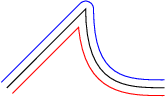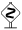# PyX — Example: drawing2/parallel.py

0.3 KB
9.6 KB
0.8 KB
0.9 KB
0.7 KB

## Paths with constant distance```from pyx import *

c = canvas.canvas()
p = path.line(0, 0, 2, 2)
p.append(path.curveto(2, 0, 3, 0, 4, 0))
c.stroke(p)
c.stroke(p, [deformer.parallel(0.2), color.rgb.blue])
c.stroke(p, [deformer.parallel(-0.2), color.rgb.red])
c.writeEPSfile("parallel")
c.writePDFfile("parallel")
c.writeSVGfile("parallel")
```

### Description

This example shows how to use the `deformer.parallel` class. It provides the path which has always constant (signed) distance to an original path.

The correct geometrical solution to the "constant distance" problem is that a corner of the original curve gets surrounded by an arc. As an alternative, you can make the deformer deviate from the strict geometrical interpretation with the argument `sharpoutercorners=1`. The resulting parallel path will then exhibit corners with the same angle as the original path does.The parallel deformer tries to find the parallel path which consists of as few path elements as possible. This is a striking feature of the parallel deformer, resulting in small EPS and PDF files, as well as in paths which can be processed further in PyX. In the example, the parallel curves for the curved right part of the original path consists of a single Bézier curve only.Note that the order of the deformer attributes is not arbitrary, since the deforming operations do not commute. If you want first to smooth and then get the parallel curve to the smoothed curve, you have to say

```[deformer.smoothed(radius), deformer.parallel(dist)]
```

since the stroke attributes are evaluated from left to right.